login  home  contents  what's new  discussion  bug reports help  links  subscribe  refresh

 Submitted by : (unknown) at: 2007-11-17T22:22:23-08:00 (15 years ago) Name : Axiom Version : default friCAS-20090114 Axiom-20050901 OpenAxiom-20091012 OpenAxiom-20110220 OpenAxiom-Release-141 Category : Axiom Aldor Interface Axiom Compiler Axiom Library Axiom Interpreter Axiom Documentation Axiom User Interface building Axiom from source lisp system MathAction Doyen CD Reduce Axiom on Windows Axiom on Linux Severity : critical serious normal minor wishlist Status : open closed rejected not reproducible fix proposed fixed somewhere duplicate need more info Optional subject :   Optional comment :

fricas
integrate(1/(1+x^4),x=%minusInfinity..%plusInfinity)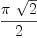(1)
Type: Union(f1: OrderedCompletion?(Expression(Integer)),...)

is obviously wrong. UPDATE: this problem is fixed in FriCAS.

fricas
integrate(1/(1+x^4),x)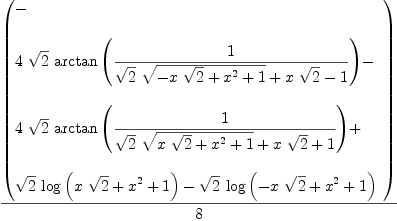(2)
Type: Union(Expression(Integer),...)

is an antiderivative only for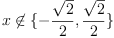. The correct antiderivative, i.e., which is defined on all of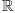can be obtained from the above expression by replacing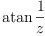with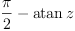. UPDATE: this problem is also fixed in FriCAS, although the result could be simpler. For example, mathematica 5.2 gives:

  In:= Integrate[1/(1+x^4), x]

Out= (-2 ArcTan[1 - Sqrt x] + 2 ArcTan[1 + Sqrt x] -

2                         2
Log[-1 + Sqrt x - x ] + Log[1 + Sqrt x + x ]) / (4 Sqrt)


However,

fricas
integrate((x^4+2*a*x^2+1)^-1, x=0..%plusInfinity, "noPole")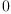(3)
Type: Union(f1: OrderedCompletion?(Expression(Integer)),...)

is wrong, also in FriCAS. However, the solution really depends on the value of a. Mathematica 5.2 gives:

  In:= Integrate[(x^4+2*a*x^2+1)^-1, {x,0,Infinity}]

2
Out= If[Im[Sqrt[-a - Sqrt[-1 + a ]]] > 0 &&

2
Im[Sqrt[-a + Sqrt[-1 + a ]]] > 0,

-I                             2                        2
(-- Pi) / (Sqrt[-a - Sqrt[-1 + a ]] Sqrt[-a + Sqrt[-1 + a ]]
2

2                          2
(Sqrt[-a - Sqrt[-1 + a ]] + Sqrt[-a + Sqrt[-1 + a ]])),

1
Integrate[---------------, {x, 0, Infinity},
2    4
1 + 2 a x  + x

Assumptions ->

2                                    2
Im[Sqrt[-a - Sqrt[-1 + a ]]] <= 0 || Im[Sqrt[-a + Sqrt[-1 + a ]]] <= 0]

]


The definite version seems to be correct:

fricas
D(integrate((x^4+2*a*x^2+1)^-1, x=0..b, "noPole"), b)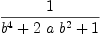(4)
Type: Expression(Integer)

another similar evaluation --kratt6, Thu, 10 Jan 2008 01:28:46 -0800 reply
The following also seems to be wrong:
fricas
ex := integrate(1/(a+x**4),x=0..%plusInfinity, "noPole")
There are no library operations named **
Use HyperDoc Browse or issue
)what op **
to learn if there is any operation containing " ** " in its name.
Cannot find a definition or applicable library operation named **
with argument type(s)
Variable(x)
PositiveInteger
Perhaps you should use "@" to indicate the required return type,
or "$" to specify which version of the function you need. eval(ex, a=1)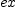(5) Type: Polynomial(Integer) compare with fricas integrate(1/(1+x**4),x=0..%plusInfinity, "noPole") There are no library operations named ** Use HyperDoc Browse or issue )what op ** to learn if there is any operation containing " ** " in its name. Cannot find a definition or applicable library operation named ** with argument type(s) Variable(x) PositiveInteger Perhaps you should use "@" to indicate the required return type, or "$" to specify which version of the function you need.

Name: #293 integrate (1/(1+x^4),x = %minusInfinity..%plusInfinity) => #293 integrate (1/(1+x^4)

Name: #293 integrate 1/(1+x)^4 => #293 integrate 1/(1+x^4)
Name: #293 integrate 1/(4x^2-1) => #293 integrate 1/(1+x^4)
Name: #293 integrate 1/(1+x^4) => #293 integrate 1x/(1+x^4)
Name: #293 integrate 1x/(1+x^4) => #293 integrate 1/(1+x^4)
Name: #293 integrate 1/(1+x^4) => #293 integrate 1/(1+x^2)